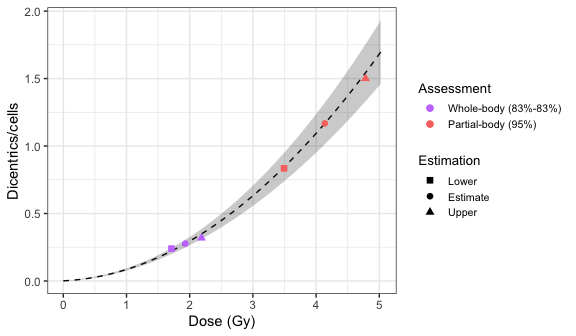# Dicentrics dose estimation

The first step is to either load the pre-calculated curve in .rds format obtained in the dose-effect fitting module or to input the curve coefficients manually. Clicking on “Preview data” will load the curve into the app and display it on the “Results” tabbed box.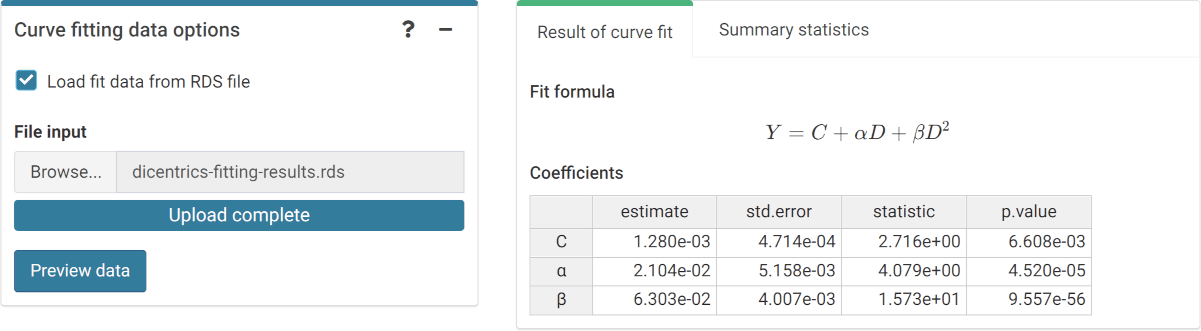‘Curve fitting data options’ box and ‘Results’ tabbed box in the dose estimation module

This step is accomplished in R by either using the results from fit() or by loading an existing .rds object via readRDS():

fit_results <- system.file("extdata", "dicentrics-fitting-results.rds",
package = "biodosetools") %>%
readRDS()
fit_results\$fit_coeffs
#>                estimate    std.error statistic      p.value
#> coeff_C     0.001280319 0.0004714055  2.715961 6.608367e-03
#> coeff_alpha 0.021038724 0.0051576170  4.079156 4.519949e-05
#> coeff_beta  0.063032534 0.0040073856 15.729091 9.557291e-56

Next we can choose to either load the case data from a file (supported formats are .csv, .dat, and .txt) or to input the data manually. Once the table is generated and filled, the “Calculate parameters” button will calculate the number of cells ($$N$$), number of aberrations ($$X$$), as well as mean ($$\bar{y}$$), error ($$\hat{\sigma}$$), dispersion index ($$\hat{\sigma}^{2}/\bar{y}$$), and $$u$$-value.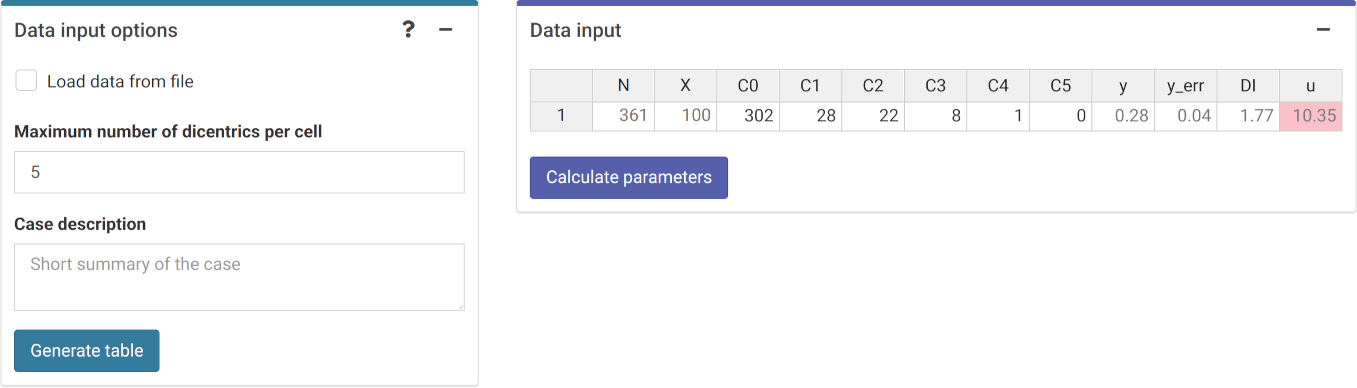‘Data input options’ and ‘Data input’ boxes in the dose estimation module

This step is accomplished in R by calling the calculate_aberr_table() function:

case_data <- system.file("extdata", "cases-data-partial.csv", package = "biodosetools") %>%
calculate_aberr_table(
type = "case",
assessment_u = 1
) %>%
dplyr::rename(y = mean, y_err = std_err)
case_data
#>     N   X  C0 C1 C2 C3 C4 C5         y      y_err       DI        u
#> 1 361 100 302 28 22  8  1  0 0.2770083 0.03683157 1.767889 10.35421

### Perform dose estimation

The final step is to select the dose estimation options. In the “Dose estimation options” box we can select type of exposure (acute, protracted, and highly protracted), type of assessment (whole-body, partial-body, or heterogeneous), and error methods for each type of assessment.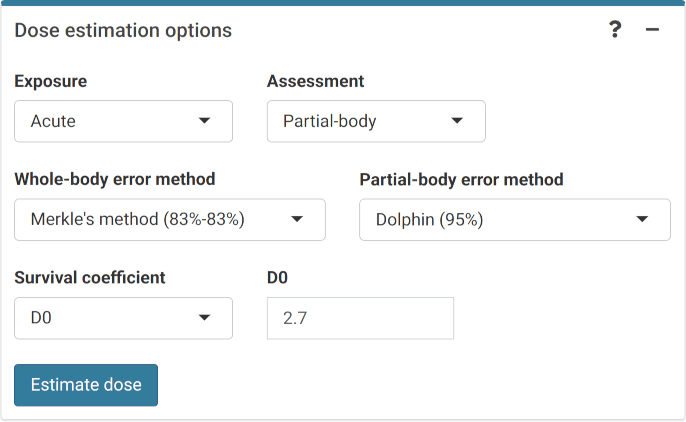‘Dose estimation options’ box in the dose estimation module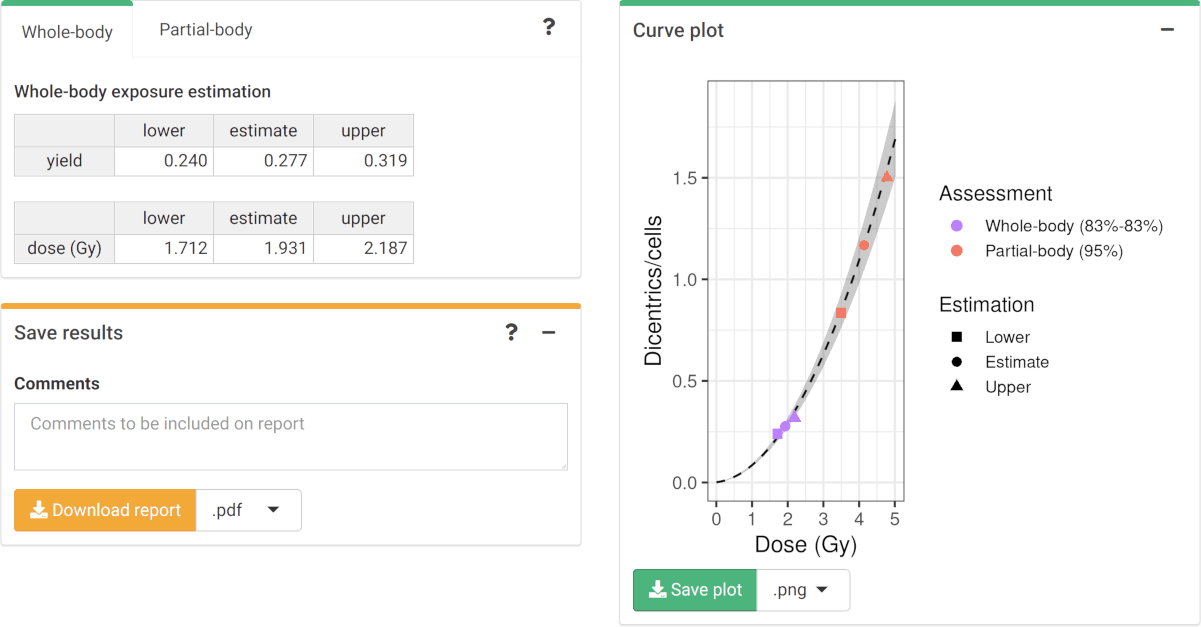‘Results’ tabbed box, ‘Curve plot’ and ‘Save results’ boxes in the dose estimation module

To perform the dose estimation in R we can call the adequate estimate_*() functions. In this example, we will use estimate_whole_body_merkle() and estimate_partial_body_dolphin(). First of all, however, we will need to load the fit coefficients and variance-covariance matrix:

fit_coeffs <- fit_results[["fit_coeffs"]]
fit_var_cov_mat <- fit_results[["fit_var_cov_mat"]]

After that is done, we can simply call estimate_whole_body_merkle() and estimate_partial_body_dolphin():

results_whole_merkle <- estimate_whole_body_merkle(
case_data,
fit_coeffs,
fit_var_cov_mat,
conf_int_yield = 0.83,
conf_int_curve = 0.83,
protracted_g_value = 1,
aberr_module = "dicentrics"
)
results_partial <- estimate_partial_body_dolphin(
case_data,
fit_coeffs,
fit_var_cov_mat,
conf_int = 0.95,
protracted_g_value = 1,
gamma = 1 / 2.7,
aberr_module = "dicentrics"
)

To visualise the estimated doses, we call the plot_estimated_dose_curve() function:

plot_estimated_dose_curve(
est_doses = list(
whole = results_whole_merkle,
partial = results_partial
),
fit_coeffs,
fit_var_cov_mat,
protracted_g_value = 1,
conf_int_curve = 0.95,
aberr_name = "Dicentrics"
)